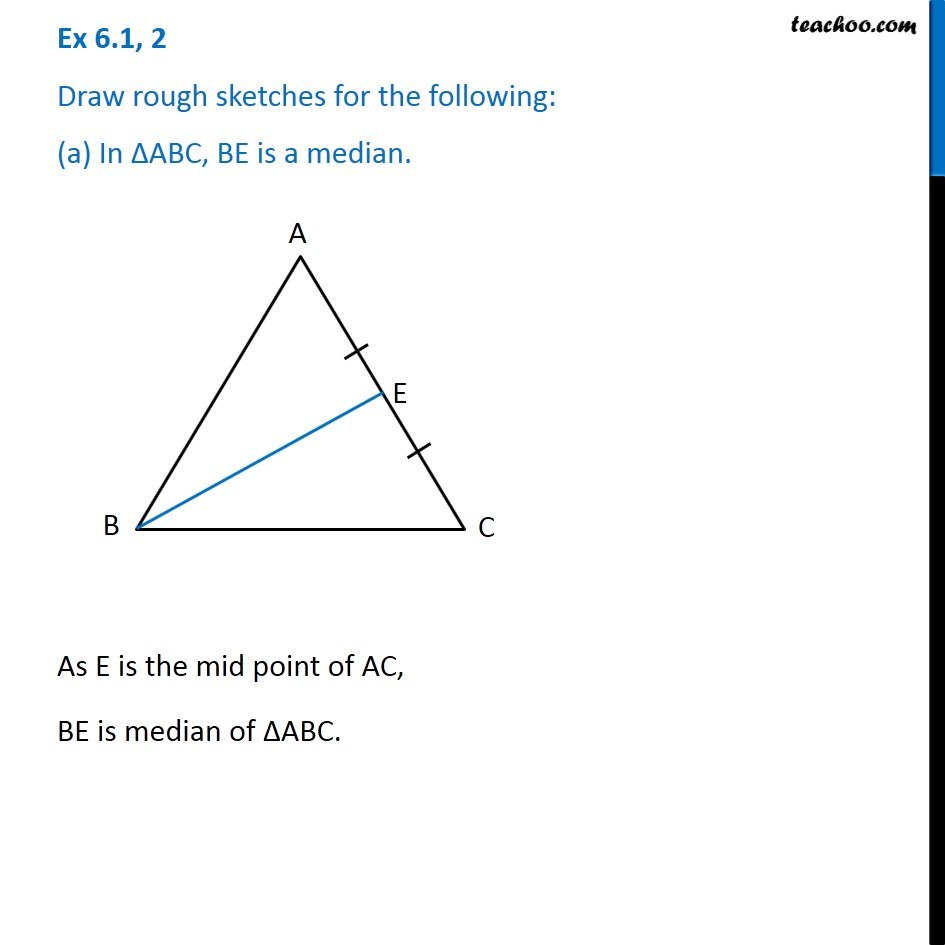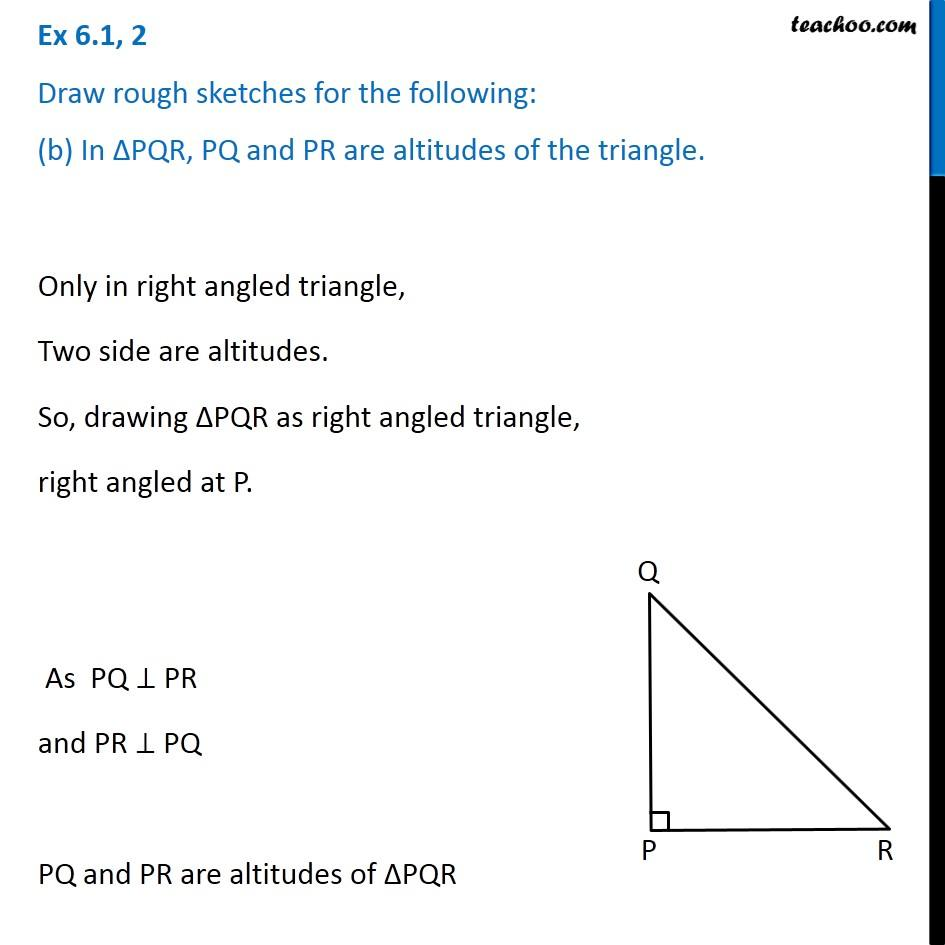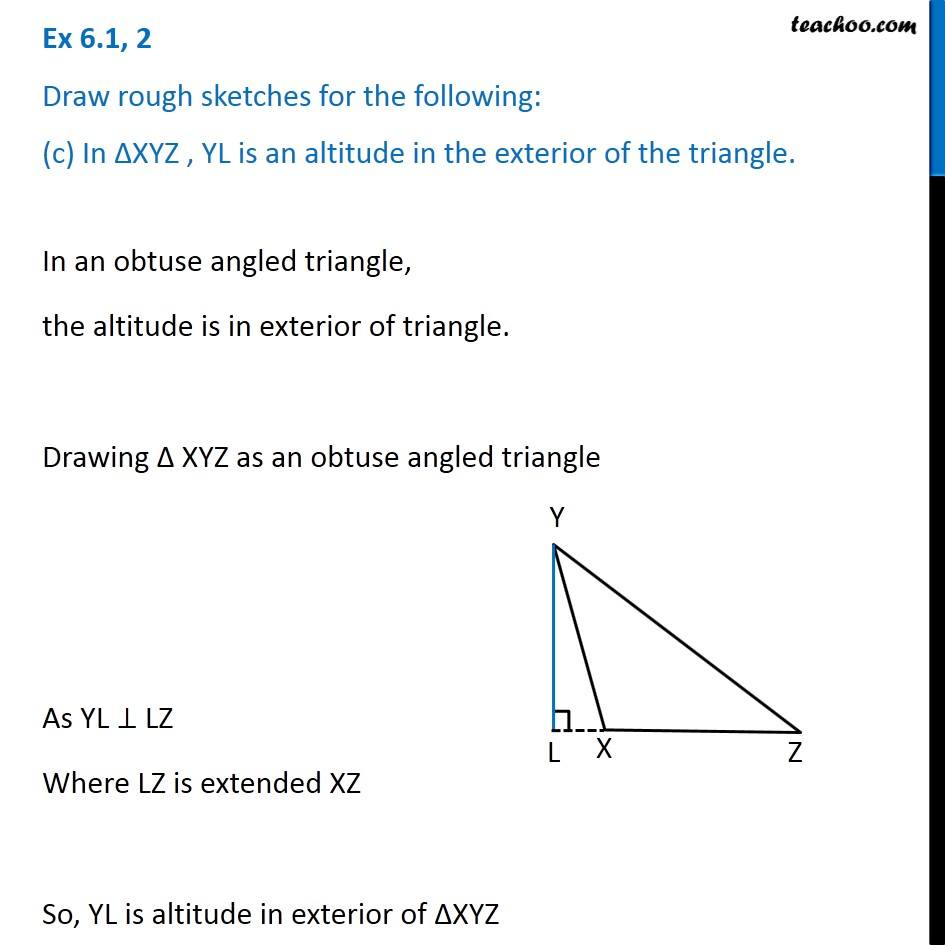Subscribe to our Youtube Channel - https://you.tube/teachoo

1. Chapter 6 Class 7 Triangle and its Properties
2. Serial order wise
3. Ex 6.1

Transcript

Ex 6.1, 2 Draw rough sketches for the following: (a) In ∆ABC, BE is a median.As E is the mid point of AC, BE is median of ∆ABC. Ex 6.1, 2 Draw rough sketches for the following: (b) In ∆PQR, PQ and PR are altitudes of the triangle. Only in right angled triangle, Two side are altitudes. So, drawing ∆PQR as right angled triangle, right angled at P. As PQ ⊥ PR and PR ⊥ PQ PQ and PR are altitudes of ∆PQR Ex 6.1, 2 Draw rough sketches for the following: (c) In ∆XYZ , YL is an altitude in the exterior of the triangle. In an obtuse angled triangle, the altitude is in exterior of triangle. Drawing Δ XYZ as an obtuse angled triangle As YL ⊥ LZ Where LZ is extended XZ So, YL is altitude in exterior of ∆XYZ

Ex 6.1